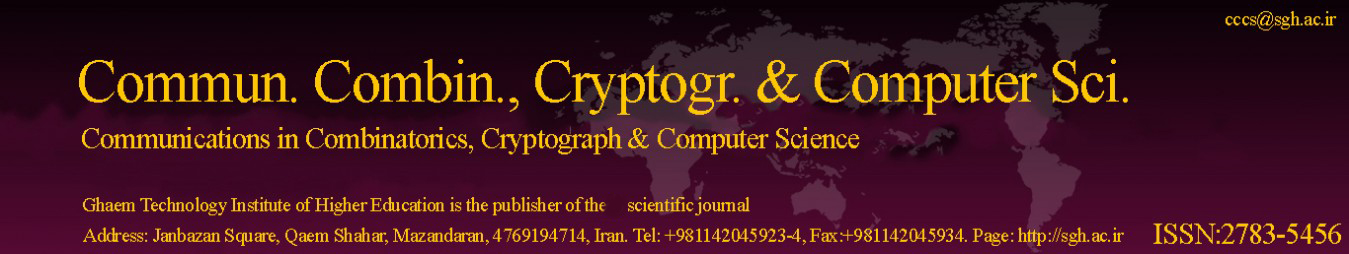Original Research Article

Article volume = 2022 and issue = 1

Pages: 55–61

Article publication Date: November 21, 2022

# An Accurate Approach to The Simulation of Nonlinear Generalized Fractional Fisher Equation

#### Hamid Mesgarani and Yones Esmaeelzade Aghdam

Department of Mathematics, Faculty of Science, Shahid Rajaee Teacher Training University, Tehran, 16785-136, I.R.Iran.

##### Abstract:

The Fisher dissemination exchange properties, as well as reaction from characteristics, make the non-linear model. The nonlinear fractional Fisher model shows up in practical physical circumstances like ultra-slow kinetics, Brownian movement of particles, anomalous diffusion, polymerases of Ribonucleic acid, deoxyribonucleic acid, continuous irregular activity, and arrangement of wave kinds. The paper considered the strategy based on the Chebyshev polynomials to get the numerical method to solve the nonlinear generalized fractional Fisher equation. The numerical scheme is developed in the following manners: at first, the semi-discrete is constructed in the temporal sense based on a linear interpolation with accuracy order $\delta ^2 t$, and secondly, the full discrete of the model is investigated. Moreover, the unconditional stability and convergence order are investigated via the numerical results. For getting of the full-discrete scheme, the spatial derivative is approched based on the shifted Chebyshev basis. In addition, the adequacy and legitimacy of the proposed modern are illustrated by means of two test.

##### Keywords:

Fisher model, Nonlinear generalized fractional Fisher equation, Chebyshev polynomials, Collocation method.

##### References:
•  H. Mesgarani, A. Beiranvand, Y. Esmaeelzade Aghdam, The impact of the Chebyshev collocation method on solutions of the time-fractional Black–Scholes, Mathematical Sciences., 15(2) (2021), 137–143. 1
•  H. Mesgarani, J. Rashidnina, Y. Esmaeelzade Aghdam, O. Nikan, The impact of Chebyshev collocation method on solutions of fractional advection–diffusion equation, International Journal of Applied and Computational Mathematics., 6(5) (2020), 6(5), 1–13. 1
•  H. Safdari, Y. Esmaeelzade Aghdam, J. Gómez-Aguilar, Shifted Chebyshev collocation of the fourth kind with convergence analysis for the space–time fractional advection-diffusion equation, Engineering with Computers., (2020), 1–12. 1
•  S. Behera, S. Saha Ray, On a wavelet-based numerical method for linear and nonlinear fractional Volterra integro- differential equations with weakly singular kernels, Computational and Applied Mathematics., 41(5) (2022), 41(5), 1–32. 1
•  M. Hashemi, D. Baleanu, On the time fractional generalized fisher equation: group similarities and analytical solutions, Communications in Theoretical Physics., 65(1) (2016), 11 pages. 1
•  Y. Liu, General solution of space fractional Fisher’s nonlinear diffusion equation, Journal of Fractional Calculus and Applications., 1(2) (2011), 6 pages. 1
•  K. M. Saad, M. Khader, J. Gómez-Aguilar, D. Baleanu, Numerical solutions of the fractional Fisher’s type equations with Atangana-Baleanu fractional derivative by using spectral collocation methods, Chaos: An Interdisciplinary Journal of Nonlinear Science., 29(2) (2019),29(2), 10 pages. 1
•  H. Yepez-Martinez, J. Reyes, I. Sosa, Analytical solutions to the fractional Fisher equation by applying the fractional sub-equation method, British Journal of Mathematics Computer Science.,4(11) (2014), 4(11), 11 pages. 1
•  M. Hashemi, D. Baleanu, On the time fractional generalized fisher equation: group similarities and analytical solutions, Communications in Theoretical Physics., 65(1) (2016), 65(1), 8 pages. 1
•  M. M. Khader, K. M. Saad, A numerical study by using the Chebyshev collocation method for a problem of biological invasion: Fractional Fisher equation, International Journal of Biomathematics., 11(08) (2018), 9 pages. 1